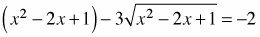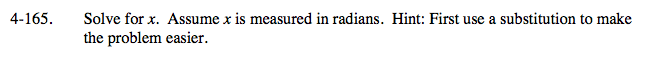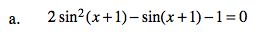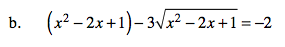### Home > CALC > Chapter 4 > Lesson 4.5.1 > Problem4-165

4-165.
1. Solve for x. Assume x is measured in radians. Hint: First use a substitution to make the problem easier. Homework Help ✎

1. 2sin2(x + 1) − sin(x + 1) − 1 = 0

2.Let U = sin(x + 1)
so 2U2U − 1 = 0

Solve for U, then substitute to solve for x.

Remember to mention ALL solutions.Let U = x2 − 2x + 1